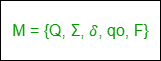Open in App
Not now

# Difference between DFA and NFA

• Difficulty Level : Easy
• Last Updated : 17 Jan, 2023

1. DFA: DFA refers to Deterministic Finite Automaton. A Finite Automata(FA) is said to be deterministic if corresponding to an input symbol, there is a single resultant state i.e. there is only one transition. A deterministic finite automata is set of five tuples represented as,Where,
Q: A non-empty finite set of states in the finite control(qo, q1, q2, …).
Σ: A non-empty finite set of input symbols.
δ: It is a transition function that takes two arguments, a state, and an input symbol, it returns a single state.
qo: It is starting state, one of the states in Q.
F: It is a non-empty set of final states/ accepting states from the set belonging to Q.

2. NFA:
NFA refers to Nondeterministic Finite Automaton. A Finite Automata(FA) is said to be non-deterministic if there is more than one possible transition from one state on the same input symbol.
A non-deterministic finite automata is also a set of five tuples and represented as,Where,
Q: A set of non empty finite states.
Σ: A set of non empty finite input symbols.
δ: It is a transition function that takes a state from Q and an input symbol from and returns a subset of Q.
qo: Initial state of NFA and member of Q.
F: A non-empty set of final states and member of Q.

Prerequisite – Finite Automata

### Difference between DFA and NFA :

My Personal Notes arrow_drop_up